# Computational Modelling on MHD Radiative Sisko Nanofluids Flow through a Nonlinearly Stretching Sheet

Computational Modelling on MHD Radiative Sisko Nanofluids Flow through a Nonlinearly Stretching Sheet

Abdullah Al-Mamun S.M. Arifuzzaman Sk. Reza-E-Rabbi Pronab Biswas Md. Shakhaoath Khan

Physics Discipline, Khulna University, Khulna-9208, Bangladesh

Centre for Infrastructure Engineering, Western Sydney University, NSW-2751, Australia

Department of Basic Sciences and Humanities, University of Asia Pacific, Dhaka-1205, Bangladesh

Mathematics Discipline, Khulna University, Khulna-9208 Bangladesh

School of Engineering, RMIT University, VIC-3001, Australia

Corresponding Author Email:
Page:
285-295
|
DOI:
https://doi.org/10.18280/ijht.370134
21 December 2018
|
Accepted:
18 February 2019
|
Published:
31 March 2019
| Citation

OPEN ACCESS

Abstract:

The boundary layer phenomena for Sisko-nano fluid flow is being observed with the effect of MHD and thermal radiation on a non-linear stretched surface. For developing a fundamental flow model, a boundary layer approximation is done, which represents time subservient momentum, concentration and energy equations. By taking the assistance of Compaq Visual Fortran, the fundamental equations are analysed by imposing a finite difference scheme explicitly. A stability and convergence study is also exhibited, and the ongoing investigation is found converged for Lewis number, Le≥0.161 and Prandtl number, Pr≥0.668. The impression of Sisko fluid parameter (A1, A2) along with diversified appropriate parameters is depicted in various flow fields. However, the developed visualisation of fluid flow is also depicted through streamlines and isotherms.

Keywords:

stability and convergence analysis, Sisko nanofluid, higher order chemical reaction, porous plate, MHD, thermal radiation

1. Introduction

Sisko fluid is a significant type of non-Newtonian fluid in the field of oil engineering, cement slurries, waterborne coating, drilling fluids, blood flow, mud and paint. Dominant Physical properties of this fluid are it gives higher viscosities at minimum share rates and lower viscosities at higher share rates, which is proposed by Sisko . Then Na and Hansen obtained a theoretical solution by using geometry and power-law model for flowing Sisko fluid between two parallel circular disks . They revealed radial distance almost linearly proportional to the pressure. By applying Homotopy Analysis Method (HAM) on Sisko fluid was showed an inverse relation between speed of the vertical belt and the non-Newtonian effect conducted by Nemati et al. . Khan and Shahzad  have done numerical investigation to understand the flow attitude of Sisko fluid on stretched surface by imposing HAM. They concluded that the effect of ascents grade of power-law index is to descent the velocity as well as boundary layer thickness. Similar investigation analysed by Munir et al. in combination with non-integral and integral data of power-law index by applying shooting technique . Sisko fluid was analysed by Hayat et al. in consider with impact of hall and heat transfer .

Additionally, Khan et al. have done a work on Sisko fluid regarding radially stretching sheet with MHD and without MHD effect [7-8]. Khan et al. addressed the flow of Sisko fluid along with forced convective heat transfer on a stretched cylinder . Moosavi et al. considered the variational iteration method (VIM) for Sisko fluid flow to scrutinize the fluid behavior through a moving belt and also in a collector . Khan et al. and Malik et al. demonstrated Cattaneo-Christov heat flux and stagnation point flow of Sisko fluid by using HAM method [11, 12]. Abbasbandy et al. studied Oldroyd-B fluid with MHD effect by applying HAM and Keller-box method . They found good uniformity between series and numerical solution in case of skin friction. Rashidi et al. devoted to a study on entropy generation by using HAM where fluid flow considered over stretching rotating disk . Many researchers addressed heat conducting phenomenon of Sisko fluid very recently [15, 16]. Choi introduced a new idea to enhance the thermal conductivity of fluid mixing with nanoparticles (Cu, Al) . Then the researchers focused on an added nanoscale particle in fluid for industrial and engineering application. Therefore, in asymmetric channel Akbar depicted the attitude of Sisko nanofluid by employing 4th and 5th order Runge-Kutta-Fehlberg scheme . Khan et al. described how the flow of Sisko nanofluid behaved on a nonlinearly stretched flat plate . They observed a monotonically increasing pattern for thermophoresis and Brownian parameters. However, for the power-law index, Prandtl number and material parameter inverse phenomena were observed. The flow attitude of 3D MHD Sisko nano and ferrofluids were examined by Raju and Sandeep on a bidirectional stretched surface . For steady Sisko nano fluid flow, Ramanaiah et al. showed temperature profile develops for improving value of thermophoresis, Brownian and thermal radiation parameters on a non-linear stretched surface . Mahmood et al. conducted a research by focusing on similar parameter with combined effect of MHD and radiation . It was depicted that thermal boundary layers developed for increasing thermophoretic parameter, but opposite phenomena was observed for Brownian parameter. The impressions of nonlinear chemical reaction along with thermal radiation were examined by Prasannakumara et al. over a nonlinear stretching sheet . They deduced an incremented character of chemical reaction parameter, thermophoresis parameter with concentration profile.

To author’s best idea, the following specific objectives of this numerical investigation have remained undone, and the objectives are:

• To investigate time subservient Sisko nanofluid flow resulting from stretched surface with the impression of heat source, mass transfer, radiation absorption along with non-linear chemical reaction.
• Mathematical formulation of the governing equations comprises unsteady momentum, energy and mass balances.
• To impose explicit finite scheme for attaining the outcomes. Also, to render a stability and convergence study for optimising flow parameters.
• To exhibit the impression of diversified parameters on different flow fields together with Cf, Nu and Sh profiles.
• To display the advanced form of fluid flow through streamlines and isotherms.
2. Governing Equations

Time subservient hydromagnetic Sisko nanofluid flow resulting from the stretched surface with the impression of heat source, radiation absorption along with non-linear chemical reaction have been studied. Here, y-axis is considered as the fluid flow direction. U0=cxs is the power law velocity. Here, s>0 stands for stretching rate. Here Tw and Cw are the fluid temperatures and concentration close to the surface whereas, T and C exhibit the same phenomena outside the boundary layer. By=B0 is the magnetic field assumed towards the flow region (Figure 1).

## 1.pngFigure 1. Flow pattern of Sisko nano-fluid

Continuity equation,

$\frac{\partial u}{\partial x}+\frac{\partial v}{\partial y}=0$ (1)

Momentum equation,

\begin{align} & \frac{\partial u}{\partial t}+u\frac{\partial u}{\partial x}+v\frac{\partial u}{\partial y}=\frac{a}{\rho }\frac{{{\partial }^{2}}u}{\partial {{y}^{2}}}-\frac{b}{\rho }\frac{\partial }{\partial y}{{\left( \frac{\partial u}{\partial y} \right)}^{n}} \\ & -\frac{\sigma B_{0}^{2}}{\rho }u+g\beta (T-{{T}_{\infty }})+g{{\beta }^{*}}(C-{{C}_{\infty }})-\frac{\upsilon }{k}u \\\end{align} (2)

Energy equation,

\frac{\partial T}{\partial t}+u\frac{\partial T}{\partial x}+v\frac{\partial T}{\partial y}=\alpha \left( \frac{{{\partial }^{2}}T}{\partial {{x}^{2}}}+\frac{{{\partial }^{2}}T}{\partial {{y}^{2}}} \right)+\frac{\upsilon }{{{c}_{p}}}{{\left( \frac{\partial u}{\partial y} \right)}^{2}}\\+\frac{{{Q}_{0}}}{\rho \,{{c}_{p}}}(T-{{T}_{\infty }})+\frac{Q_{1}^{*}}{\rho \,{{c}_{p}}}(C-{{C}_{\infty }})-\frac{1}{\rho {{c}_{p}}}\frac{\partial {{q}_{r}}}{\partial y}\\+\frac{{{D}_{m}}{{\kappa }_{T}}}{{{c}_{s}}\,{{c}_{p}}}\frac{{{\partial }^{2}}C}{\partial {{y}^{2}}}+\Gamma \left\{ \begin{align} & {{D}_{B}}\left( \frac{\partial T}{\partial x}\frac{\partial C}{\partial x}+\frac{\partial T}{\partial y}\frac{\partial C}{\partial y} \right) \\ & +\frac{{{D}_{T}}}{{{T}_{\infty }}}\left[ {{\left( \frac{\partial T}{\partial x} \right)}^{2}}+{{\left( \frac{\partial T}{\partial y} \right)}^{2}} \right] \\\end{align} \right\} (3)

Concentration equation,

\begin{align} & \frac{\partial C}{\partial t}+u\frac{\partial C}{\partial x}+v\frac{\partial C}{\partial y}={{D}_{B}}\left( \frac{{{\partial }^{2}}C}{\partial {{x}^{2}}}+\frac{{{\partial }^{2}}C}{\partial {{y}^{2}}} \right) \\ & +\frac{{{D}_{T}}}{{{T}_{\infty }}}\left( \frac{{{\partial }^{2}}T}{\partial {{x}^{2}}}+\frac{{{\partial }^{2}}T}{\partial {{y}^{2}}} \right)-{{K}_{c}}{{(C-{{C}_{\infty }})}^{P}} \\\end{align} (4)

with boundary conditions,

$u={{U}_{0}}=c{{x}^{s}},\,T={{T}_{w}},\,\,C={{C}_{w}}\,\,\,\,\text{at}\,\,\,\,\,\,y=0$

$u\to 0,\,T\to {{T}_{\infty }},\,C\to {{C}_{\infty }}\,\,\text{at}\,\,\,\,\,\,y\to \infty \,$

Here, Q0 is the heat source, Q1* denotes the radiation absorption, Kc species chemical reaction and p is the order. The Rosseland approximation is exhibited as, ${{q}_{r}}=-\frac{4{{\sigma }_{s}}}{3{{k}_{e}}}\frac{\partial {{T}^{4}}}{\partial y}$. Then the equation (3) becomes,

## equation_5.png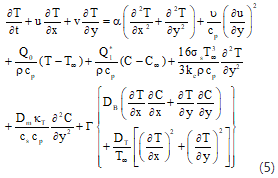For solving the fundamental equations (1)-(5) the dimensionless quantities are adopted as,

\begin{align} & X=\frac{x{{U}_{0}}}{\upsilon },Y=\frac{y{{U}_{0}}}{\upsilon },\,U=\frac{u}{{{U}_{0}}},V=\frac{v}{{{U}_{0}}},\,\tau =\frac{tU_{0}^{2}}{\upsilon },\,\, \\ & \theta =\frac{T-{{T}_{\infty }}}{{{T}_{w}}-{{T}_{\infty }}},\,\phi =\frac{C-{{C}_{\infty }}}{{{C}_{w}}-{{C}_{\infty }}}. \\\end{align}

Hence non-dimensional forms are obtained as,

Continuity equation,

$\frac{\partial U}{\partial X}+\frac{\partial V}{\partial Y}=0$ (6)

Momentum equation,

\begin{align} & \frac{\partial U}{\partial \tau }+U\frac{\partial U}{\partial X}+V\frac{\partial U}{\partial Y}=\frac{{{\partial }^{2}}U}{\partial {{Y}^{2}}}\left[ {{A}_{1}}-{{A}_{2}}n{{(\frac{\partial U}{\partial Y})}^{n-1}} \right] \\ & +{{G}_{r}}\theta +{{G}_{c}}\phi -MU-\frac{U}{{{D}_{a}}} \\ \end{align} (7)

Energy equation,

\begin{align} & \frac{\partial \theta }{\partial \tau }+U\frac{\partial \theta }{\partial X}+V\frac{\partial \theta }{\partial Y}=\frac{1}{{{P}_{r}}}\left( 1+\frac{16R}{3} \right)\frac{{{\partial }^{2}}\theta }{\partial {{Y}^{2}}}+\frac{1}{{{P}_{r}}}\frac{{{\partial }^{2}}\theta }{\partial {{X}^{2}}} \\ & +Q\theta +{{Q}_{1}}\phi +{{E}_{c}}{{\left( \frac{\partial U}{\partial Y} \right)}^{2}}+{{D}_{u}}\frac{{{\partial }^{2}}\phi }{\partial {{Y}^{2}}} \\ & +{{N}_{b}}\left( \frac{\partial \theta }{\partial X}\frac{\partial \phi }{\partial X}+\frac{\partial \theta }{\partial Y}\frac{\partial \phi }{\partial Y} \right)+{{N}_{t}}\left[ {{\left( \frac{\partial \phi }{\partial X} \right)}^{2}}+{{\left( \frac{\partial \theta }{\partial X} \right)}^{2}} \right] \\\end{align} (8)

Concentration equation,

\begin{align} & \frac{\partial \phi }{\partial \tau }+U\frac{\partial \phi }{\partial X}+V\frac{\partial \phi }{\partial Y}=\frac{1}{{{L}_{e}}}\left( \frac{{{\partial }^{2}}\phi }{\partial {{X}^{2}}}+\frac{{{\partial }^{2}}\phi }{\partial {{Y}^{2}}} \right) \\ & +\frac{{{N}_{t}}}{{{N}_{b}}{{L}_{e}}}\left( \frac{{{\partial }^{2}}\theta }{\partial {{X}^{2}}}+\frac{{{\partial }^{2}}\theta }{\partial {{Y}^{2}}} \right)-{{K}_{c}}{{\phi }^{P}} \\\end{align} (9)

With the conditions,

\begin{align} & U=1,\,\,\,\theta =1,\,\,\varphi =1\,\,\,\,\,\text{at}\,\,\,\,\,\,y=0 \\ & U=0,\,\,\theta =0,\,\varphi =0\,\,\,\,\,\text{at}\,\,\,\,\,y\to \infty \, \\\end{align}

where, Magnetic parameter, $M\text{=}\frac{\sigma '{{B}_{o}}^{2}v}{\rho{{U}_{o}}^{2}}$, mass Grashof number, ${{G}_{c}}=\frac{g\beta *({{c}_{w}}-{{c}_{\infty }})v}{U_{_{o}}^{2}}$, Grashof number, ${{G}_{r}}=\frac{g\beta ({{T}_{w}}-{{T}_{\infty }})}{U_{0}^{3}}$, Darcy number, ${{D}_{a}}=\frac{k'U_{0}^{2}}{{{v}^{2}}}$, Prandtl number, ${{\operatorname{P}}_{r}}=\frac{\rho {{c}_{p}}v}{k}$, Eckert number, ${{E}_{\text{c}}}=\frac{U_{0}^{2}}{{{c}_{p}}({{T}_{w}}-{{T}_{\infty }})}$, radiation parameter, $R=\frac{\sigma T_{\infty }^{3}}{{{k}_{e}}\kappa }$, heat source parameter, $Q=\frac{{{Q}_{o}}v}{U_{\text{o}}^{2}\rho {{c}_{p}}}$, radiation absorption parameter, ${{Q}_{1}}=\frac{Q_{1}^{*}v}{U_{\text{o}}^{2}\rho {{c}_{p}}}\frac{({{C}_{w}}-{{C}_{\infty }})}{({{T}_{w}}-{{T}_{\infty }})}$, Dufour number, ${{D}_{u}}=\frac{{{D}_{m}}kT}{{{c}_{s}}{{c}_{p}}v}\frac{({{C}_{w}}-{{C}_{\infty }})}{({{T}_{w}}-{{T}_{\infty }})}$, Lewis number, $L_e=\frac{v}{D_m}$, Sisko fluid parameter, $A_1=\frac{a}{ρυ}$ and ${{A}_{\text{2}}}\text{=}\frac{bU_{0}^{2n-2}}{\rho {{v}^{n}}}$, Brownian parameter, ${{N}_{b}}\text{=}\frac{r{{D}_{B}}({{C}_{w}}-{{C}_{\infty }})}{v}$, thermophoresis parameter ${{N}_{t}}\text{=}\frac{r{{D}_{T}}}{{{T}_{\infty }}v}({{T}_{w}}-{{T}_{\infty }})$, chemical reaction, ${{K}_{c}}\text{=}\frac{v{{K}_{c}}({{C}_{w}}-{{C}_{\infty }})}{U_{0}^{2}}$ and Order of chemical reaction = P.

Here, equation (6) is satisfied by stream function ψ and associated with velocity component as, $U=\frac{∂ψ}{∂Y}$ and $V=\frac{∂ψ}{∂X}$.

3. Section Shear Stress, Nusselt and Sherwood Number

The impression of different parameters on local and average shear stress is being computed from the velocity. Here, Local and average shear stresses are $\tau A=\mu \int{{{(\frac{\partial u}{\partial y})}_{y=0}}}dx$  respectively which are proportionate to ${{(\frac{\partial u}{\partial y})}_{y=0}}$ and $\int _{0}^{100}{{(\frac{\partial u}{\partial y})}_{y=0}}dX$ respectively. Now, the influence of different parameters has been examined from the temperature field on local as well as average heat transfer coefficient. In this case, the local and average Nusselt number, ${{N}_{uL}}=\mu {{(-\frac{\partial T}{\partial y})}_{y=0}}$ and ${{N}_{uA}}=\mu {{(-\frac{\partial T}{\partial y})}_{y=0}}dx$ are also proportionate to ${{(-\frac{\partial \theta }{\partial Y})}_{y=0}}$ and $\int_{0}^{100}{{{(-\frac{\partial \theta }{\partial Y})}_{y=0}}}$. However, from concentric field, the average and local mass transfer have been analysed with the impact of diversified parameters such that average ${{S}_{hA}}=\mu \int{{{(-\frac{\partial C}{\partial y})}_{y=0}}}$ and local ${{S}_{hL}}=\mu {{(-\frac{\partial C}{\partial y})}_{y=0}}$  mass transfer rate proportionate $\int_{0}^{100}{{{(-\frac{\partial \varphi }{\partial Y})}_{Y=0}}}dX$ and ${{(-\frac{\partial \varphi }{\partial Y})}_{Y=0}}$ respectively.

4. Numerical Simulation

Equations (6)-(9) are being solved by imposing explicit finite scheme within the given boundary criterion. A rectangular shape flow region is chosen in which the grid lines are distributed parallel to x and y-axes (Figure 2). For the existing problem, it is adopted as Ymax = 20, which represents the length of the plate. It changes from o to 20 as Y→∞. However, the grid spaces are also considered as, m = 100 and n = 200 respectively and Δτ = 0.005. Now, we adopt the following equations by employing explicit finite scheme.

## 2.pngFigure 2. Illustration of grid spacing

$\frac{{{U}_{i,j}}-{{U}_{i,j-1}}}{\Delta X}+\frac{{{V}_{i,j+1}}-{{V}_{i,j}}}{\Delta Y}=0$ (10)

\begin{align} & \frac{U_{i,j}^{'}-{{U}_{i,j}}}{\Delta \tau }+{{U}_{i,j}}\frac{{{U}_{i,j}}-{{U}_{i-1,j}}}{\Delta X}+{{V}_{i,j}}\frac{{{U}_{i,j+1}}-{{U}_{i,j}}}{\Delta Y}={{G}_{r}}{{\theta }_{i,j}} \\ & +{{G}_{c}}{{\phi }_{i,j}}\,-(M+\frac{1}{{{D}_{a}}}){{U}_{i,j}}+\frac{{{U}_{i,j+1}}-2{{U}_{i,j}}+{{U}_{i,j-1}}}{{{(\Delta Y)}^{2}}} \\ & \left\{ {{A}_{1}}-{{A}_{2}}n{{\left( \frac{{{U}_{i,j+1}}-{{U}_{i,j}}}{\Delta Y} \right)}^{n-1}} \right\} \\\end{align} (11)

\begin{align} & \frac{\theta _{i,j}^{'}-{{\theta }_{i,j}}}{\Delta \tau }+{{U}_{i,j}}\frac{{{\theta }_{i,j}}-{{\theta }_{i-1,j}}}{\Delta X}+{{V}_{i,j}}\frac{{{\theta }_{i,j+1}}-{{\theta }_{i,j}}}{\Delta Y}=Q{{\theta }_{i,j}} \\ & +\frac{1}{{{P}_{r}}}(1+\frac{16}{3}R)\frac{{{\theta }_{i,j+1}}-2{{\theta }_{i,j}}+{{\theta }_{i,j-1}}}{{{(\Delta Y)}^{2}}}+{{Q}_{1}}{{\phi }_{i,j}} \\ & +\frac{1}{{{P}_{r}}}\left( \frac{{{\theta }_{i+1,j}}-2{{\theta }_{i,j}}+{{\theta }_{i-1,j}}}{{{(\Delta X)}^{2}}} \right)+{{D}_{u}}\frac{{{\phi }_{i,j+1}}-2{{\phi }_{i,j}}+{{\phi }_{i,j-1}}}{{{(\Delta Y)}^{2}}} \\ & +{{E}_{c}}{{\left( \frac{{{U}_{i,j+1}}-{{U}_{i,j}}}{\Delta Y} \right)}^{2}}+{{N}_{t}}\left\{ {{\left( \frac{{{\phi }_{i,j}}-{{\phi }_{i-1,j}}}{\Delta X} \right)}^{2}}+{{\left( \frac{{{\theta }_{i,j+1}}-{{\theta }_{i,j}}}{\Delta Y} \right)}^{2}} \right\} \\ & +{{N}_{b}}\left( \frac{{{\theta }_{i,j}}-{{\theta }_{i-1,j}}}{\Delta X}.\frac{{{\varphi }_{i,j}}-{{\varphi }_{i-1,j}}}{\Delta X}+\frac{{{\theta }_{i,j+1}}-{{\theta }_{i,j}}}{\Delta Y}.\frac{{{\varphi }_{i,j+1}}-{{\varphi }_{i,j}}}{\Delta Y} \right) \\\end{align} (12)

## equation_13.png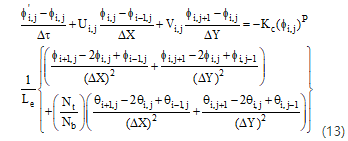With boundary conditions,

$U_{i,0}^{n}=1,\,\,\theta _{i,0}^{n}=1,\,\,\phi _{i,0}^{n}=1\\U_{i,L}^{n}=0,\,\,\theta _{i,L}^{n}=0,\,\,\phi _{i,L}^{n}=0$ where, $L\to \infty$

Here, i=j= grid points along X and Y axes and $\tau =n\Delta \tau$, where, n= positive number.

5. Stability and Convergence Study

Due to the implementation of explicit finite scheme the ongoing investigation demands the study of stability and convergence test. It won’t be necessary to use equation (6) because Δτ doesn’t appear on it. At an arbitrary time the Fourier transformation gives the following equations.

\left. \begin{align} & U:\psi (\tau ){{e}^{i\alpha X}}{{e}^{i\beta Y}} \\ & \theta :\theta (\tau ){{e}^{i\alpha X}}{{e}^{i\beta Y}} \\ & \varphi :\varphi (\tau ){{e}^{i\alpha X}}{{e}^{i\beta Y}} \\\end{align} \right\} (14)

And after a time step we adopt,

\left. \begin{align} & U:{{\psi }^{'}}(\tau ){{e}^{i\alpha X}}{{e}^{i\beta Y}} \\ & \theta :{{\theta }^{'}}(\tau ){{e}^{i\alpha X}}{{e}^{i\beta Y}} \\ & \varphi :{{\varphi }^{'}}(\tau ){{e}^{i\alpha X}}{{e}^{i\beta Y}} \\\end{align} \right\} (15)

Substituting Equation (14) and (15) to Equations (11)-(13) we attain,

\begin{align} & {{\psi }^{'}}=\psi +\Delta \tau [{{G}_{r}}\theta +{{G}_{c}}\varphi -(M+\frac{1}{{{D}_{a}}})\psi +\frac{2(\cos \beta \Delta Y-1)}{{{(\Delta Y)}^{2}}}\psi \\ & -\frac{U(1-{{e}^{i\alpha \Delta X}})}{\Delta X}\psi -\frac{V({{e}^{i\beta \Delta Y}}-1)}{\Delta Y}\psi \\ & -\frac{2\psi (\cos \beta \Delta Y-1)}{{{(\Delta Y)}^{2}}}\left\{ {{A}_{1}}-{{A}_{2}}n\psi {{\left( \frac{({{e}^{i\beta \Delta Y}}-1)}{\Delta Y} \right)}^{n-1}} \right\}] \\\end{align}\\herefore {\psi }'={{A}_{1}}\psi +{{A}_{2}}\theta +{{A}_{3}}\varphi (16)

where, ${{A}_{2}}=\Delta \tau {{G}_{r}}$ and ${{A}_{3}}=\Delta \tau {{G}_{c}}$ and

\begin{align} & {{A}_{1}}=1+\Delta \tau \frac{2(\cos \beta \Delta Y-1)}{{{(\Delta Y)}^{2}}}\left\{ {{A}_{1}}-{{A}_{2}}n{{\left( \frac{({{e}^{i\beta \Delta Y}}-1)}{\Delta Y} \right)}^{n-1}} \right\} \\ & -\Delta \tau (M+\frac{1}{{{D}_{a}}})-\frac{U\Delta \tau (1-{{e}^{i\alpha \Delta X}})}{\Delta X}-\frac{V\Delta \tau ({{e}^{i\beta \Delta Y}}-1)}{\Delta Y} \\\end{align}

For temperature equation,

## equation_17.png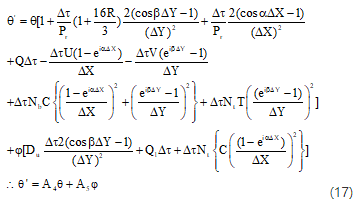where,

${{A}_{5}}={{D}_{u}}\frac{\Delta \tau 2(\cos \beta \Delta Y-1)}{{{(\Delta Y)}^{2}}}+{{Q}_{1}}\Delta \tau +\Delta \tau {{N}_{t}}\left\{ C{{\left( \frac{(1-{{e}^{i\alpha \Delta X}})}{\Delta X} \right)}^{2}} \right\}$

And

## equation.png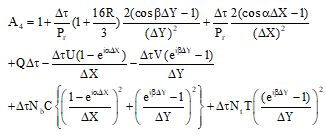For the concentration equation,

## equation_18.pngwhere, \begin{align} & {{A}_{6}}=1+\frac{\Delta \tau }{{{L}_{e}}}\left\{ \frac{2(\cos \beta \Delta X-1)}{{{(\Delta X)}^{2}}}+\frac{2(\cos \beta \Delta Y-1)}{{{(\Delta Y)}^{2}}} \right\}-\Delta \tau {{K}_{r}} \\ & -\frac{U(1-{{e}^{i\alpha \Delta X}})}{\Delta X}-\frac{V({{e}^{i\beta \Delta Y}}-1)}{\Delta Y} \\\end{align} and ${{A}_{7}}=\frac{{{N}_{t}}}{{{N}_{b}}}\left\{ \frac{2(\cos \beta \Delta X-1)}{{{(\Delta X)}^{2}}}+\frac{2(\cos \beta \Delta Y-1)}{{{(\Delta Y)}^{2}}} \right\}$

Equation (16)-(18) can be expressed in matrix notation,

$\left[ \begin{matrix} \psi ' \\ \theta ' \\ \varphi ' \\\end{matrix} \right]=\left[ \begin{matrix} {{A}_{1}} & {{A}_{2}} & {{A}_{3}} \\ 0 & {{A}_{4}} & {{A}_{5}} \\ 0 & {{A}_{7}} & {{A}_{6}} \\\end{matrix} \right]\left[ \begin{matrix} \psi \\ \theta \\ \varphi \\\end{matrix} \right]$  i.e. $\eta '=T'\eta$

Diversified data Δτ→0 makes the investigation critical. Hence, for Δτ→0 we adopt, A2→0,A3→0,A5→0  and  A7→0.

$\therefore T'=\left[ \begin{matrix} {{A}_{1}} & 0 & 0 \\ 0 & {{A}_{4}} & 0 \\ 0 & 0 & {{A}_{6}} \\\end{matrix} \right]$

So, the Eigenvalues are attained as A11,A42  and A63  which satisfies, |A1 |≤1,|A4 |≤1  and |A6|≤1

Now taking,

\begin{align} & {{a}_{1}}=\Delta \tau ,\,{{b}_{1}}=U\frac{\Delta \tau }{\Delta X},\,{{c}_{1}}=\left| -V \right|\frac{\Delta \tau }{\Delta Y}\,\,,{{d}_{1}}=2\frac{\Delta \tau }{{{\left( \Delta X \right)}^{2}}}\text{,}{{\text{e}}_{1}}=2\frac{\Delta \tau }{{{\left( \Delta Y \right)}^{2}}} \\ & \alpha \Delta Y=m\pi ,\alpha \Delta X=n\pi ,U=\,\text{positive}\,\,\text{and}\,\,V=\,\text{negative}. \\\end{align}

Keeping in mind the above-considered things the stability criterion of this investigation can be achieved after simplification as,

\begin{align} & U\frac{\Delta \tau }{\Delta X}+V\frac{\Delta \tau }{\Delta Y}+\frac{2}{{{P}_{r}}}(1+\frac{16}{3}R)\frac{\Delta \tau }{{{\left( \Delta Y \right)}^{2}}}+\frac{2}{{{P}_{r}}}\frac{\Delta \tau }{{{\left( \Delta X \right)}^{2}}}+\frac{\Delta \tau \,Q}{2} \\ & +4{{N}_{b}}C\left( \frac{\Delta \tau }{{{\left( \Delta X \right)}^{2}}}+\frac{\Delta \tau }{{{\left( \Delta Y \right)}^{2}}} \right)+2{{N}_{t}}T\frac{\Delta \tau }{{{\left( \Delta Y \right)}^{2}}}\le 1 \\\end{align}

and $U\frac{\Delta \tau }{\Delta X}+V\frac{\Delta \tau }{\Delta X}+\frac{2}{{{L}_{e}}}\left( \frac{\Delta \tau }{{{\left( \Delta X \right)}^{2}}}+\frac{\Delta \tau }{{{\left( \Delta Y \right)}^{2}}} \right)+\frac{\Delta \tau \,{{K}_{c}}}{2}\le 1$

For U=V=T=C=0,Δτ=0.005,ΔY=0.25 and ΔX=0.20, the existing problem converged at Le≥0.161 and Pr≥0.668.

6. Results and Discussion

The flow character of hydromagnetic naturally convective Sisko fluid through a perpendicular non-linear stretching sheet with the appearance of nanoparticles is being studied numerically. The numerical computation for different flow fields is depicted by FORTRAN. The time-independent resolutions have executed up to non-dimensional time $\tau =30$. Graphical results are analysed using physical parameters, such as Gr=8.00, Da=1.60, Gm=2.00, M=1.20, A1=1.20, A2=0.60, L=1, Pr=2, Du=0.03, R=0.30, Ec=0.002, Q=1.40, Q1=0.06, Le=8.00, Nt=0.1, Nb=0.1 and Kc=0.50.

## 3.png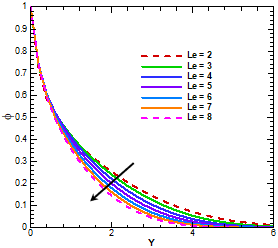Figure 3. Variation of concentration profiles due to Le

Figure 3 depicts the impact of Lewis number, Le on concentric fields. By escalating the data of Le indicate the decline of concentric fields with initial thin line spread out by the difference of large value from value of Y=1.1, where this behaviour is attributed to the effect Le (inversely proportional to DB) as Le rises when DB decreased. Figure 4 demonstrates the impact Lewis number on Sherwood number where the plot is shown downward pattern of Sh concerning Lewis number. The impression of Dufour number, Du, is drafted on temperature profiles in Figure 5.

## 4.png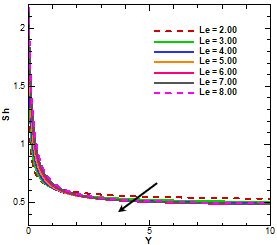Figure 4. Variation of Sherwood number due to Le

## 5.pngFigure 5. Variation of temperature profiles due to Du

It can be perceived that for the growing values of Du noticed the increase of temperature distribution from initial to the point of Y=1.6. After this point, the decremented pattern is shown to the end with insignificant differences. The upshots of thermophoresis, Nt, and Brownian, Nb, parameters on temperature fields are established in Figures 6 and 7. Furthermore, the numerical change of Nb and Nt concerning temperature by percentage is shown in Table 2 were small change occurred in case of Nb instead of significant change in Nt.

The fundamental causes behind are thermophoresis parameter directly proportional to temperature difference and Brownian motion increase by the effect of incremented concentration. The contrasts of Prandtl number, Pr, are described in Figure 8. It is witnessed that initially enlarged data of temperature profile become reverse at the point near about Y=2.

Table 1. Comparison of skin friction coefficient with Prasanna kumara et al.  when, Nr=R=1.5, θw=1.2, Nb=Nt=0.45, Le=10, , γ=K_c=0.1, Pr=6.2

 Linear Stretching Sheet (n=1) Nonlinear Stretching Sheet (n=3) A, A1, A2 Q S Skin Friction  Present Study Skin Friction  Present Study 0.5 0.3 0.5 1.1583 1.1623 0.9486 0.9684 1 0.3 0.5 1.3417 1.1418 1.1259 1.1339 2 0.3 0.5 1.6582 1.1695 1.4526 1.1506 0.5 0 0.5 0.9530 0.9936 0.7567 0.8019 0.5 0.3 0.5 1.1583 1.1590 0.9486 0.9713 0.5 0.6 0.5 1.3360 1.3452 1.1248 1.1302 0.5 0.3 0 0.8559 0.8831 0.5365 0.6348 0.5 0.3 0.5 1.1583 1.1632 0.9486 0.9684

Table 2. Variation of Curve for diversified data of Nb and Nt in Figure 6 and Figure 7 at Y=4

 Nb $\theta$ Increase Nt θ Increase 1.10 0.26876 0.20 0.36071 1.50 0.26881 0.005 % 0.25 0.38820 2.749 % 1.90 0.26889 0.008 % 0.30 0.41705 2.885 % 2.40 0.26898 0.009 % 0.40 0.47878 6.173 %

## 6.png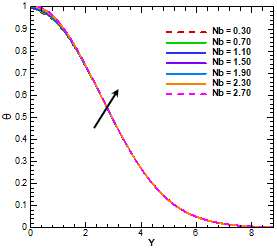Figure 6. Variation of temperature profiles due to Nb

Then it is established the reduction of temperature distribution by the impact of incremented Pr. Because of high Prandtl number creates low thermal conductivity. To explore the character of Q (heat source), on temperature profiles, Figure 9 is plotted. It is asserted that initially temperature profile incremented with rising of Q but finally decreased. Before the value of Y=2.4, it shows incremented with difference of Q=0.20 and Q=0.40 about 119.156% at Y=2.00 but finally decreased by 1.014% at Y=6.00. Figure 10 displays the impression of Q1 (radiation absorption), upon temperature profiles for diversified rising values of Q1. It is seen that temperature of Sisko nanofluid drops as Q1 upsurge.

## 7.png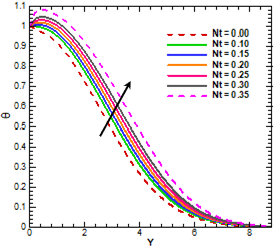Figure 7. Variation of temperature profiles due to Nt

## 8.png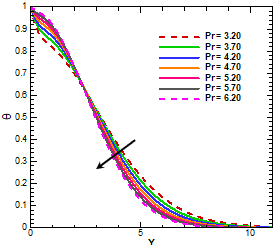Figure 8. Variation of temperature profiles due to Pr

## 9.pngFigure 9. Variation of temperature profiles due to Q

## 10.pngFigure 10. Variation of temperature profiles due to Q1

Figure 11 depicts chemical reaction impact on concentration profiles. On the evidence of these figures, it is investigated that the values of Kc are raised, the concentration profile depresses. This occurs because the incremented of Kc enhance the chemical reaction and consequently the concentration profile reduces.

Furthermore, the velocity distribution for various data of Sisko fluid parameters A1 andA2 is organised in Figures 12 and 13. Here, the velocity distribution decremented with growing value only for A1 but reversed for A2. Both figures exhibit their change in about 50% before twisting at a point nearly Y=1.8. However, the Darcy number, Da, impact on velocity profile is sketched in Figure 14. The fluid velocity is found increasing with increased Da.

## 11.png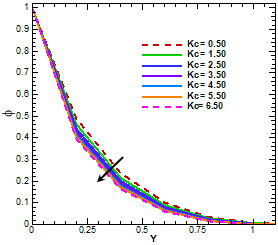Figure 11. Variation of concentration profiles due to Kr

## 12.png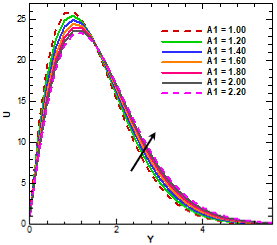Figure 12. Variation of velocity profiles due to A1

## 13.pngFigure 13. Variation of velocity profiles due to A2

Table 3. Variation of Curve for different value of A1 and A2 in Figure 12 and Figure 13 at Y=3

 A1 U Increase A2 U Decrease 1.00 4.65671 0.60 5.17792 1.20 5.17792 52.121 % 0.80 4.65671 52.121 % 1.40 5.65896 48.104 % 1.00 4.11640 54.031 % 1.60 6.09619 43.723 % 1.20 3.66987 44.653 %

The change of percentage for Y=3, from range of value Da= 2.00 to 2.60 is described numerically at Table 4. Finally, the change becomes indistinct for their similar value at far away from plate. The variation of radiation parameter, R, is displayed in Figure 15. It is anticipated that fluid temperature enhances with ascending values of R. It is claimed that the radiation parameter is related with third power of the temperature. Initially, downward pattern changes after Y=1.6 until the end of plate which detects numerically at point Y=4 in Table 5. From Figure 16, it is examined that an increment in Ra initially created reduction pattern but finally incremented with Nusselt number.

Table 4. Variation of Curve for different value of Da in Figure 14 at Y=3

 Da U Increment in Percentage 2.00 1.80661 2.20 1.84366 3.705 % 2.40 1.87461 3.095 % 2.60 1.90071 2.610 %

## 14.png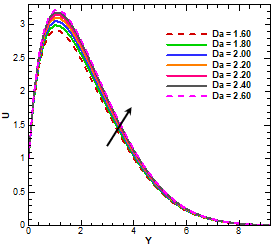Figure 14. Variation of velocity profiles due to Da

## 15.png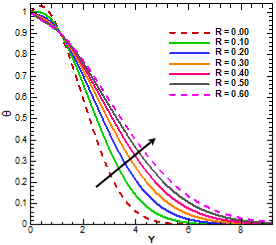Figure 15. Variation of temperature profiles due to R

## 16.png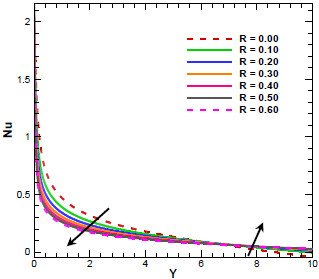Figure 16. Variation of Nusselt number due to R

Table 5. Variation of curve for different R in Figure 15 and Figure 16 at Y=4

 Figure 15 Figure 16 R θ Increase R Nu Decrease 0.00 0.06244 0.00 0.17853 0.10 0.13794 7.550 % 0.10 0.15646 2.207 % 0.20 0.20878 7.084 % 0.20 0.14102 1.544 % 0.30 0.26921 6.043 % 0.30 0.12945 1.157 %

In case of Nusselt number change of percentage from the value of R=0.00 to R=0.30 is displayed in Table 5 which continue at the point of Y=6.2. Furthermore, Figure 17, we elucidated the dleomination of magnetic parameter, M, on velocity. Here we detect a rising value of M indicates the descending value of velocity profile.

## 17.png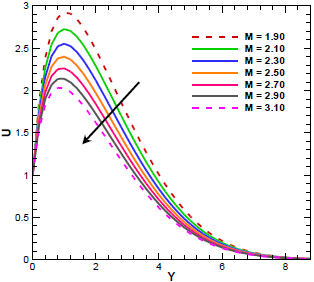Figure 17. Variation of velocity profiles due to M

The fact behind this, the appearance of magnetic field develops Lorentz force. This force retracts fluid flows. The decremented pattern becomes more distinct by numerically (Table 6). That’s shown differences between a line to another line in Figure 17 with respect considering grid by percentage. The impact of M on skin friction is given in Figure 18. It is evident from this Figure, the value of M enhances by decreasing skin friction. In table 6 represents the change in the percentage of M from 1.90 to 2.50.

Table 6. Variation of curve for different M in Figure 17 and Figure 18 at Y = 3

 Figure 17 Figure 18 M U Increase M U Decrease 1.90 1.00763 1.90 0.60374 2.10 0.91484 7.550 % 2.10 0.56139 2.207 % 2.30 0.83544 7.084 % 2.30 0.52236 1.544 % 2.50 0.76786 6.043 % 2.50 0.48618 1.157 %

## 18.png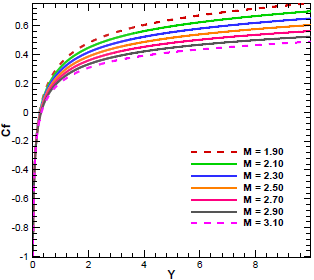Figure 18. Variation of Skin friction due to M

It indicates the apparent features of different M from Y = 1.1 to the end of the plate. The analysis of isotherms and streamlines are depicted in Figures 19 to 21 for exhibiting the advanced visualisation of fluid fields. In Figures. 19 and 20, isotherms for different M with different view (line and flood) is portrayed, which indicate thermal boundary layers increase due to with developing magnetic parameter, M. To reveal the distinct visualisation of fluid flow, the streamlined flow is illustrated in Figures 21 and 22 for increasing values of M. It can be perceived by sketching tangent on the velocity direction of fluids. Here it is experienced that, momentum boundary layers get suppress for increasing magnetic parameter.

## 19.png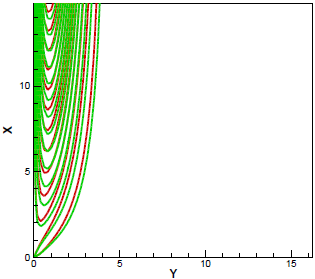Figure 19. Isotherms view for M=1.20 (red solid line) and M=2.00 (green solid line)

## 20.png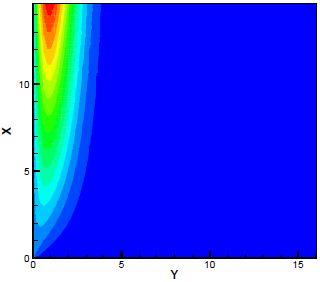Figure 20. Isotherms flood view for different M

## 21.pngFigure 21. Streamline line view for M=1.20 (red solid line) and M=2.00 (green solid line)

## 22.png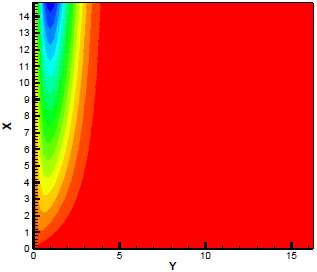Figure 22. Streamline flood view for different M

7. Conclusion

Computational modelling of Sisko fluid with nanoparticle moving to a porous stretching sheet with non-linear chemical reaction is being analysed. The following results are noticed after conducting the complete study:

• The concentration distribution decreasing for the improving behaviour of chemical reaction and Lewis number.
• The temperature profile decremented with ascending of Dufour number, Prandtl number, heat source and radiation absorption parameters but enhances by thermophoresis, Brownian and thermal radiation parameters.
• The behaviour of velocity distribution decrease for magnetic parameter and one Sisko fluid parameter and increase for Darcy number and other Sisko fluid parameters.
• Nusselt number increase for increasing radiation parameter.
• The Skin friction distribution is falling for upsurge value of the magnetic parameter.
• Sherwood number is showed descending pattern with enhancing Lewis number.
Nomenclature
 A, A1, A2 Sisko fluid parameter (-) B○ magnetic component, (Wb m-2) Cf skin-friction, (-) Cp specific heat at constant pressure, (J kg-1 K-1) Da Darcy number, (-) DB The Brownian diffusion coefficient, (-) Du Dufour number, (-) Ec Eckert number, (-) Gr Grashof number, (-) Gc modified Grashof number, (-) K/ the permeability of the porous medium, (-) ke mean absorption coefficient Kr chemical reaction parameter, (-) Le Lewis number, (-) Nb The Brownian parameter, (-) Nt thermophoresis parameter, (-) Nu local Nusselt number, (-) Pr Prandtl number, (-) Q heat source parameter, (-) qr unidirectional radiative heat flux, (kg m-2) Q1 radiation absorption, (-) R radiation parameter (-) Sh Sherwood number, (-) T Fluid temperature, (K) Tw The temperature at the plate surface, (K) T∞ ambient temperature as y tends to infinity, (K) U○ uniform velocity u, v velocity components x, y Cartesian coordinates Greek symbols β thermal expansion coefficient β* concentration expansion co-efficient κ thermal conductivity, (Wm-1 K-1) μ dynamic viscosities ν kinematic viscosity, (m2 s-1) ρ the density of the fluid, (kg m-3) σ' electric conductivity σs Stefan-Boltzmann constant, 5.6697 × 10-8 (W/m2K4)
References

 Sisko AW. (1958). The flow of lubricating greases. Industrial and Engineering Chemistry 50: 1789-1792. https://doi.org/10.1021/ie50588a042

 Na TY, Hansen AG. (1967). Radial flow of viscous non-Newtonian fluids between disks. International journal of Non-Linear Mechanics 2: 261-273. https://doi.org/10.1016/0020-7462(67)90027-3

 Nemati H, Ghanbarpour M, Hajibabayi M, Hemmatnezhad. (2009). Thin film flow of non-Newtonian fluids on a vertical moving belt using Homotopy Analysis Method. Journal of Engineering Science and Technology Review 2(1): 118-122. https://doi.org/10.1002/fld.2320

 Khan M, Shahzad A. (2013). On boundary layer flow of a Sisko fluid over a stretching sheet. Quaestiones Mathematicae, 36: 137-151. https://doi.org/10.2989/16073606.2013.779971

 Munir A, Shahzad A, Khan M. (2014). Forced convective heat transfer in boundary layer flow of Sisko fluid over a nonlinear stretching sheet. PLoS ONE 9(6): e100056. https://doi.org/10.1371/journal.pone.0100056

 Hayat T, Maqbool K, Asghar S. (2009). Hall and heat transfer effects on the steady flow of a Sisko fluid. A Journal of Physical Sciences 64(12): 769-782. https://doi.org/10.1515/zna-2009-1201

 Khan M, Malik R, Munir A, Shahzad A. (2016). MHD flow and heat transfer of Sisko fluid over a radially stretching sheet with convective boundary conditions. Journal of the Brazilian Society of Mechanical Science and Engineering 38(4): 1279-1289. https://doi.org/10.1007/s40430-015-0437-y

 Khan M, Malik R, Munir A. (2015). Mixed convective heat transfer to Sisko fluid over a radially stretching sheet in the presence of convective boundary conditions. AIP Advances 5: 087178. https://doi.org/10.1063/1.4929832

 Khan M, Malik R. (2015). Forced convective heat transfer to Sisko fluid flow past a stretching cylinder. AIP Advances 5: 127202. https://doi.org/10.1063/1.4937346

 Moosavi M, Momeni M, Tavangar T, Mohammadyari R, Rahimi-Esbo M. (2016). Variational iteration method for flow of non-Newtonian fluid on a moving belt and in a collector. Alexandria Engineering Journal 55: 1775-1783. https://doi.org/10.1016/j.aej.2016.03.033

 Khan M, Malik R, Hussain M. (2016). Nonlinear radiative heat transfer to stagnation-point flow of Sisko fluid past a stretching cylinder AIP Advances 6: 055315. https://doi.org/10.1063/1.4950946

 Malik R, Khan M, Mushtaq M. (2016). Cattaneo-Christov heat flux model for Sisko fluid flow past a permeable non-linearly stretching cylinder. Journal of Molecular Liquids 222: 430-434. https://doi.org/10.1016/j.molliq.2016.07.040

 Abbasbandy S, Hayat T, Alsaedi A, Rashidi MM. (2014). Numerical and analytical solutions for Falknar-Skan flow of MHD Oldroyd-B fluid. International Journal of Numerical Methods for Heat and Fluid Flow 24(2): 390-401. https://doi.org/10.1108/HFF-05-2012-0096

 Rashidi MM, Ali M, Freidoonimehr N, Nazari F. (2013). Parametric analysis and optimization of entropy generation in unsteady MHD flow over a stretching rotating disk using artificial neural network and particle swarm optimization algorithm. Energy 55: 497-510. https://doi.org/10.1016/j.energy.2013.01.036

 Khan M, Ahmad L, Khan WA, Alshomranic AS, Alzahranic AK, Alghamdi MSA. (2017). 3D Sisko fluid flow with Cattaneo-Christov heat flux model and heterogeneous-homogeneous reactions: A numerical study Journal of Molecular Liquids 238: 19-26. https://doi.org/10.1016/j.molliq.2017.04.059

 Malik R, Khan M, Shafiq A, Mushtaq M, Hussain M. (2017). An analysis of Cattaneo-Christov double-diffusion model for Sisko fluid flow with velocity slip. Results in Physics 7: 2458-2469. https://doi.org/10.1016/j.rinp.2017.03.027

 Choi SUS. (1998). Enhancing thermal conductivity of fluids with nanoparticles. In: Siginer DA, Wang HP (eds) Developments and applications of non-Newtonian flows. 66. ASME, New York, 99-105.

 Akbar NS. (2014). Peristaltic Sisko nano fluid in an asymmetric channel. Applied Nanoscience 4: 663-673. https://doi.org/10.1007/s13204-013-0205-1

 Khan M, Malik R, Munir A, Khan WA. (2015). Flow and heat transfer to Sisko nanofluid over a nonlinear stretching sheet. PLoS ONE 10(5): e0125683. https://doi.org/10.1371/journal.pone.0125683

 Raju CSK, Sandeep N. (2016). Heat and mass transfer in 3D non-Newtonian nano and ferro fluids over a bidirectional stretching surface. International Journal of Engineering Research in Africa 21: 33-51.

 Ramanaiah GV, Babu MS, Lavanya ML. (2016). Convective heat and mass transfer flow of Sisko nanofluid past a nonlinear stretching sheet with thermal radiation. International Refereed Journal of Engineering and Science 5(2): 17-37. https://doi.org/10.1166/jon.2019.1620

 Mahmood T, Iqbal Z, Ahmed J, Shahzad A, Khan M. (2017). Combined effects of magnetohydrodynamics and radiation on nano Sisko fluid towards a nonlinear stretching sheet. Results in Physics 7: 2458-2469. https://doi.org/10.1016/j.rinp.2017.05.003

 Prasannakumara BC, Gireesha BJ, Krisnamurthy MR, Kumar KG. (2017). MHD flow and nonlinear radiative heat transfer of Sisko nanofluid over a nonlinear stretching sheet. Informatics in Medicine Unlocked 9: 123-132. https://doi.org/10.1016/j.imu.2017.07.006

 Hayat T, Kara AH, Mommoniat E. (2005). The unsteady flow of a fourth-grade fluid past a porous plate. Mathematical and Computer Modeling 41: 1347-1353. https://doi.org/10.1016/j.mcm.2004.01.010

 Rashad AM, Chamkha AJ, Abdou MMM. (2013). Mixed convection flow of non-Newtonian fluid from vertical surface saturated in a porous medium filled with a nanofluid. Journal of Applied Fluid Mechanics 6(2): 301-309.

 Rashidi MM, Hayat T, Erfani E, Mohimanian Pour SA, Hendi AA. (2011). Simultaneous effects of partial slip and thermal-diffusion and diffusion-thermo on steady MHD convective flow due to a rotating disk. Communications in Nonlinear Science and Numerical Simulation 16: 4303-4317. https://doi.org/10.1016/j.cnsns.2011.03.015

 Raju CSK, Babu MJ, Sandeep N, Sugunamma V, Reddy JVR. (2015). Radiation and soret effects of MHD nanofluid flow over a moving vertical moving plate in porous medium. Chemical and Process Engineering Research 30: 9-21.

 Rashidi MM, Kavyani N, Abelman S. (2013). Investigation of entropy generation in MHD and slip flow over a rotating porous disk with variable properties. International Journal of Heat and Mass Transfer 70: 892-917. https://doi.org/10.1016/j.ijheatmasstransfer.2013.11.058

 Pandey AK, Kumar M. (2017). Natural convection and thermal radiation influence on nanofluid flow over a stretching cylinder in a porous medium with viscous dissipation. Alexandria Engineering Journal 55(1): 55-62. https://doi.org/10.1016/j.aej.2016.08.035

 Arifuzzaman SM, Khan MS, Hossain KE, Islam MS, Akter S, Roy R. (2017). Chemically reactive viscoelastic fluid flow in presence of nano particle through porous stretching sheet. Frontiers in Heat and Mass Transfer 9(5): 2151-8629. https://doi.org/10.5098/hmt.9.5

 Bég OA, Khan MS, Karim I, Alam MM, Ferdows M. (2014). Explicit Numerical study of unsteady hydromagnetic mixed convective nanofluid flow from an exponentially stretching sheet in porous media. Applied Nanoscience 4(8): 943-957. https://doi.org/10.1007/s13204-013-0275-0

 Khan MS, Rahman MM, Arifuzzaman SM, Biswas P, Karim I. (2017). Williamson fluid flow behaviour of MHD convective and radiative Cattaneo-Christov heat flux type over a linearly stretched surface with heat generation, viscous dissipation and thermal-diffusion. Frontiers in Heat and Mass Transfer 9(15): 1-11. http://dx.doi.org/10.5098/hmt.9.15

 Kasaeian A, Axarian RD, Mahian O, Kolsi L, Chamkha AJ, Wongwises S, Pop I. (2017). Nanofluid flow and heat transfer in porous media: A review of the latest developments. International Journal of Heat and Mass Transfer 107: 778-791. https://doi.org/10.1016/j.ijheatmasstransfer.2016.11.074

 Biswas P, Arifuzzaman SM, KarimI, Khan MS. (2017). Impacts of magnetic field and radiation absorption on mixed convective Jeffrey nano fluid flow over vertical stretching sheet with stability and convergence analysis. Journal of Nanofluids 6(6): 1082-1095. https://doi.org/10.1166/jon.2017.1407

 Arifuzzaman SM, Mehedi MFU, Mamun AA, Khan MS. (2018). Magnetohydrodynamic micropolar fluid flow in presence of nanoparticles through porous plate: A numerical study. International Journal of Heat and Technology 36(3): 936-948. https://doi.org/10.18280/ijht.360321

 Arifuzzaman SM, Khan MS, Islam MS, Islam, MM, Rana BMJ, Biswas P, Ahmed SF. (2017). MHD Maxwell fluid flow in presence of nano-particle through a vertical porous-plate with heat-generation, radiation absorption and chemical reaction. Frontiers in Heat and Mass Transfer 9: 25. http://dx.doi.org/10.5098/hmt.9.25

 Arifuzzaman SM, Khan MS, Mehedi MFU, Rana BMJ, Biswas P, Ahmed SF. (2018), Chemically reactive and naturally convective high speed MHD fluid flow through an oscillatory vertical porous plate with heat and radiation absorption effect. Engineering Science and Technology, an International Journal 21(2): 215-228. https://doi.org/10.1016/j.jestch.2018.03.004

 Biswas P, Arifuzzaman SM, Khan MS. (2018). Effects of periodic magnetic field on 2D optically dense gray nano fluid over a vertical plate: a computational EFDM study with SCA. Journal of Nanofluids 7: 1-10. https://doi.org/10.1166/jon.2018.1434

 Arifuzzaman SM, Khan MS, Mamun AA, Rabbi SRE, Biswas P, Karim I. (2019). Hydrodynamic stability and heat and mass transfer flow analysis of MHD radiative fourth-grade fluid through porous plate with chemical reaction. Journal of King Saud University – Science (Article in Press). https://doi.org/10.1016/j.jksus.2018.12.009

 Rabbi SRE, Arifuzzaman SM, Sarkar T, Khan MS, Ahmmed SF. (2018). Explicit finite difference analysis of an unsteady MHD flow of a chemically reacting casson fluid past a stretching sheet with brownian motion and thermophoresis effects. Journal of King Saud University – Science (Article in press). https://doi.org/10.1016/j.jksus.2018.10.017

 Arifuzzaman SM, Rana BMJ, Ahmed R, Ahmmed SF. (2017). Cross diffusion and MHD effect on a HighOrder chemically reactive micropolar fluid of naturally convective heat and mass transfer past through an infinite vertical porous medium with a constant heat sink. AIP Conference Proceedings 1851: 020006. http://dx.doi.org/10.1063/1.4984635

 Arifuzzaman SM, Biswas P, Mehedi MFU, Ahmmed SF, Khan MS. (2018). Analysis of unsteady boundary layer viscoelastic nanofluid flow through a vertical porous plate with thermal radiation and periodic magnetic field. Journal of Nanofluids 7(6): 1022-1029. http://dx.doi.org/10.1166/jon.2018.1547